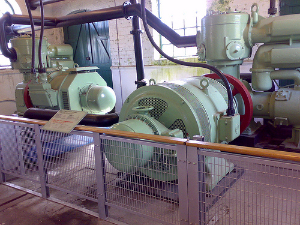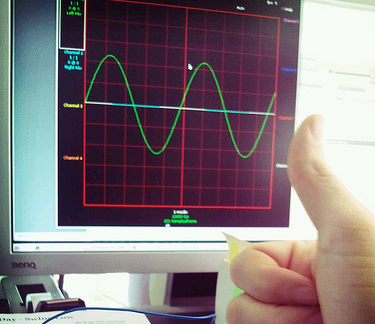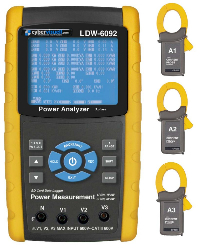# How Can Electricians Make Money With Power Factor Correction?Air compressors typically need power factor correction.. Photo courtesy of espinr(CC Attribution)

Power factor is a measurement that tells us how effectively electrical power is being used by equipment. If a site has a low power factor, it can cause extra loading on the electrical supply systems – on the customer site as well as in the power grid and power station.

These days many electrical utilities actually charge their larger customers more if they have a low power factor, as it costs the utility more to supply them. So it makes a lot of sense to find out what the power factor is on commercial and industrial sites. Then power factor correction equipment can be installed to correct the problem, resulting in big savings on electricity bills.AC Voltages cycle up and down.. Photo courtesy of wstryder(CC Attribution)

## Understanding power factor

In AC electrical systems, the voltage cycles up and down (positive and negative) 50 or 60 times per second. Consider a system with a simple electrical load such as a light bulb. In the AC cycling of voltage, when the voltage is maximum, the current flow should also be maximum, and when the voltage passes through zero, the current should also be zero. In this system, the amount of real power used by the light bulb is exactly equal to voltage x current (using RMS measurements).

But now let’s consider an electric motor as a load, with coiled windings. These coils create a magnetic field, which interacts back with the electrical current. This causes the maximum current to flow at a different time, when the voltage is not maximum. The result is that the amount of real power converted to movement by the motor is no longer equal to voltage x current (RMS). It is less than this. This means with a low power factor, the motor does less physical work, but the current flow in the electrical circuits is still high.

So we now have 2 measurements:

• Real power (this is the amount of power that has turned into physical work, such as movement, light or heat, by the light bulb or motor);
• Apparent power (this is a calculation of RMS current x RMS voltage).

The Power Factor is the ratio of Real Power to Apparent Power. It is always a number between 0 and 1.

Hence in the example of the motor, say it draws 10 amps at a voltage of 10 volts AC, and say the motor has a power factor of 0.5. Then the Real Power will be 10 x 10 x 0.5 = 50 Watts which is the amount of physical rotating power the motor has. The Apparent Power will be 10 x 10 = 100 VA (volt-amps). Hence the electrical supply circuitry will still have to supply 10 Amps of current, even though the motor is only actually outputting 50 watts of turning power. If we can install Power Factor Correction to correct the power factor in the electrical circuit, then the current would reduce to 5 amps RMS and hence it will be easier on the electricity supply. The motor output would still remain at 50 watts though.Photo courtesy of Gordon Werner(CC ShareALike)

## What causes a low power factor?

Low power factor is usually caused by “reactive loads”. This is mostly equipment that uses electrical coils, such as motors, pumps, air compressors, machine tools, and transformers.

## How does power factor correction work?

Power factor correction normally consists of a bank of capacitors, which are best installed near the equipment that has a low power factor. The capacitors store energy during the AC cycle and return it at the right time to bring the current flow into the correct timing. This restores the power factor close to the ideal value of 1.

## How to find out the power factor?

The easiest way to find out the power factor is to use a 3 phase power data logger that measures power factor. Clamp the current transformers around the cables supplying each piece of equipment, and attach the voltage probes to the mains. The instrument will give an immediate readout of power factor. For equipment that varies its operation over time, it is best to set the instrument to record the power parameters over a period of time such as a week, then download and check the data to find out the average power factor over time.

You can then use this information to calculate how much extra it is costing to run this equipment due to the power factor, and you can choose a suitable power factor correction device.

##How does this benefit me as an electrical contractor?

As an electrical contractor, particularly if you work on commercial and industrial sites, you can earn extra money and save your customers money with power factor correction. This only applies to customers who are billed according to their kVA usage, rather than kW.

All you need to do is use a 3 phase power data logger to measure the power factor on your customer’s sites, then you can immediately see if there is an issue. The data logger will also show kVA and kW used by the equipment.

You can calculate the potential savings on their electricity bill from power factor correction as follows:

Potential Annual Savings in Dollars = (kVA – kW) x unitcost x 24 x 365 / 100

where unitcost is the electricity price in cents per kVAh. If the equipment is not running 24 hours per day then you can change it from 24 to the number of hours of use. Similarly if it’s not running 365 days per year then change this figure.

Once you demonstrate how much your customer can save, they will thank you for installing power factor correction devices to save them money.About Chris Dobbie

Chris Dobbie is an experienced Systems Engineer focused on Industrial Technology. As the owner of Esis (Sydney, Australia) he has had exposure to a wide range of industrial electronic equipment in a variety of applications, and also has extensive system design and C/C++ programming experience. Contact Chris if you want to chat about your project!